##### Common Core Math For Parents For Dummies with Videos Online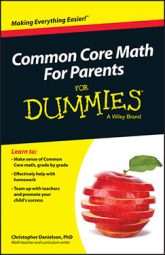In Common Core geometry, fourth graders work with fractions of a full rotation — halves, quarters, and so on. They also work with angle measurement in degrees, where a degree is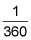of a full rotation. They use protractors to measure angles to the nearest whole degree.

In geometry, two rays with a common vertex form an angle, as you can see in the figure.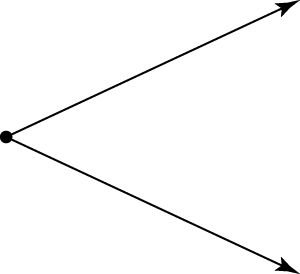An angle is two rays with a common vertex.

A ray is a portion of a line. A ray has one endpoint (the vertex) and extends infinitely in one direction.

This mathematical definition is usually not that useful for fourth graders (nor even for many 16‐year‐old geometry students). Fortunately, fourth graders have many experiences with angles that they can build on in the classroom. In particular, fourth graders know about turns. They have spun on the playground, they have tried to turn all the way around while jumping in the air, they have sat on swivel stools, they know that owls can turn their heads much farther than people can, and so on.

When you measure an angle, you measure the size of the turn between the two rays.

Here's an example. Gather a few neighborhood children and do some turns through various angles. Can you turn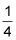of a full rotation to the right? Can you turn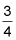of a full rotation to the left? If you can do each of these from the same starting direction, the result is the same. But the angles are different. The first one is a 90-degree angle; the second one is a 270-degree angle.

Theturn to the right technically has a measure of negative 90 degrees, but in the fourth grade, this distinction isn't important.

Fourth graders pay attention to fractions of a full rotation — halves, quarters, and so on. They also develop angle measurement in degrees, where a degree isof a full rotation. They use protractors to measure angles to the nearest whole degree.